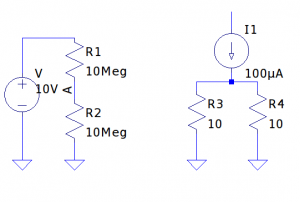Electrometer is a piece of highly specialized electronic test equipment. Most of us would naturally associate it with low-level measurements (e.g. low current pA, fA and high resistance GΩ measurements) only, but the situations under which you might need to use an electrometer are actually far more common then you might have thought. In this post I will discuss a couple of situations where using an electrometer is crucial. And at the end of the post I included a video for some demonstrations.

For those who does low level measurements, Keithley‘s Low Level Measurements Handbook (now its 7th edition) has a wealth of information and is a must read. By definition provided in the handbook, an electrometer is an instrument that is able to:

• Measure current lower than 10nA
• Measure resistance higher than 10GΩ
• Minimize circuit loading (e.g. Measure voltage from a source resistance of 100MΩ or higher and measure current with minimum voltage burden)
• Measure charge
• Measure signals at or near Johnson noise limitations

Even for measurement ranges well within a typical multimeter, there are many situations where an electrometer may be needed in order to obtain accurate results.

Consider the voltage divider circuit on the left below:Since most of the multimeters have an input impedance of roughly 10MΩ, we would get erroneous readings if we were to probe the circuit directly due to the loading effect of our meter impedance. While in this case, we could calculate the theoretical value of the divider voltage by taking into consideration the resistance of the meter, in other situations this might not be feasible. For instance, if we were to measure the biasing voltage of a JFET circuit by measuring the gate voltage, due to the loading effect of our multimeter, we may have changed the operating region of the circuit and thus cannot simply deduce the theoretical value.

In this case, an electrometer would come in handy due to its very high input impedance (Keithley 614 for instance has an input impedance of 50TΩ in its voltage mode). Some multimeters like the Keithley 196, has a high input impedance range for certain ranges which can also be used for this type of measurements.

Another example is shown in the second circuit to the right above. Here we wanted to measure the current flowing through one of the two paralleled resistors. While in this case 50 µA flows through each side, which is not a terribly small current, we nevertheless can not use a standard multimeter to measure this current due to meter’s high burden voltage. In this example, the voltage drop across the 10Ω resistor is only 1mV and you will need to use an electrometer (or feedback ammeter) to obtain accurately measurement result. If you don’t have an electrometer or nanoammeter, a simple current adapter should be suffice for current in the nA range.

In the following video, I demonstrated the above scenarios and also included a couple of low current measurement experiments towards the end.